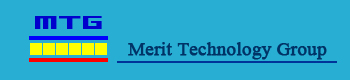Home About Us Products Production Equipments Peltier Technology Order Online Contact UsPeltier Technology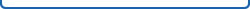Performance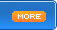In the application of refrigeration devices, to further understand its performance, The fact refrigeration from the cold end of the heat absorption around Q, there are two, one is the joule heat Qj; Another is the sensible heat conduction.Electricity from the internal components have passed on Joule heat, Joule heat reached the half-cold, and the other half spread hot end, heat conduction from the hot end reached the end of the cold.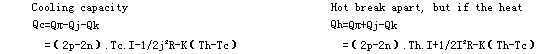Type, said one pair of R galvanic resistivity of the total K is the total thermal conductivity. From the above two formulas, we can see that the importation of electric power is just breaks the heat-hot and cold-heat absorption of the poor, This is the "heat pump" a : Qh-Qc=I2R=PMolecular-Qc I 2 = R = P          From a drawn-Galvanic the hot end of the heat released to the importation of Molecular electric power production with the cold end of the cold up and come to the opposite end of the Cold cooling capacity Qc hot end to the heat emitted from electric power and the importation of the poor.          Qh=P+QcQ2 = P + Qc          Qc=Qh-PQ2 = Qc-P          Uncooled largest power calculation method.          A.1 Th at 27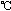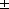1,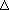T=0,I=Imax .A.1 hot-temperature Th 271, the temperature ofT = 0, I = Imax time.          Qcmax(W) according to formula (1): Qcmax largest refrigeration power (W) by the formula          (1) Calculations :          Qcmax=0.07NI????(1)Qcmax = 0.07NI (1)          In the formula: (A).Where : N -- Components of a few, I -- the largest thermoelectric device current (A)          A.2 If the surface temperature of the hot 3 ~ 40, Qcmax largest refrigeration power (W) shall formula (2) be amended.          Qcmax | Th= Qcmax*[1+0.0042(Th--27)]          (2)Qcmax | Th = Qcmax * [1 +0.0042 (Th -- 27)] (2)          (W),Where : Qcmax --- Th hot surface temperature = 271maximum cooling power (W),          Qcmax | Th (W).Qcmax | Th -- hot surface temperature Th -- 3 ~ 40when the measured temperature of the greatest cooling power (W).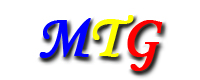2001-2009 COPYRIGHT MERIT TECHNOLOGY GROUP.ALL RIGHTS RESERVED.    粤ICP备09185414号-1       Address：Luogang Industry Park, Buji Town,Longgang District,Shenzhen. 518112 China Sales Hot-line：0086-755-28583356        Fax：0086-755-28580892        Mob：0086-13622328755 Email：info@merittegroup.com        Website：http://www.merittegroup.com merittegroup@126.com     Post office entrance